# Finite-dimensional representation

(diff) ← Older revision | Latest revision (diff) | Newer revision → (diff)
A unitary finite-dimensional representation is a direct sum of irreducible unitary finite-dimensional representations. The intersection of the kernels of the continuous homomorphisms of a topological groupcoincides with the intersection of the kernels of the irreducible unitary finite-dimensional representations of; if this set contains only the identity of, then there is a continuous monomorphism frominto some compact group, andis said to be imbeddable in a compact group, or to be a maximally almost-periodic group (a MAP-group). Ifis a MAP-group, then the family of matrix entries of irreducible finite-dimensional representations ofseparates points in. Commutative groups and compact groups are MAP-groups; a connected locally compact group is a MAP-group if and only if it is the direct product of a connected compact group and(see ). A MAP-group can have infinite-dimensional irreducible unitary representations and need not be a group of type 1. Every continuous irreducible unitary representation of a locally compact groupis finite-dimensional if and only ifis a projective limit of finite extensions of groupsof the form, where,andare closed subgroups ofsuch thatis isomorphic to,is compact andis a discrete group that is central in; a sufficient condition is that the quotient group ofby its centre be compact. Moreover, for many locally compact groups (in particular, for non-compact simple Lie groups), the only irreducible unitary finite-dimensional representation is the trivial representation.
Non-unitary finite-dimensional representations of topological groups have been classified (up to equivalence) only for special groups; in particular, forand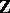, where the problem of describing finite-dimensional representations is solved by reducing matrices to Jordan form, and — in the case of— is related to the theory of ordinary differential equations with constant coefficients, in that the solution space of such an equation is a finite-dimensional invariant subspace of the regular representation ofin the space of continuous functions on. In addition, the finite-dimensional representations of connected semi-simple Lie groups are known. More precisely, these are direct sums of irreducible finite-dimensional representations that can be described as follows. Ifis a semi-simple complex Lie group andis a maximal compact subgroup, then every continuous irreducible unitary representationofin a spacecan be extended: 1) to an irreducible representationofinwhose matrix entries are analytic functions on; and 2) to an irreducible representationofwhose matrix entries are complex conjugates of analytic functions on;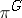and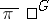are determined uniquely by. The tensor product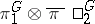is an irreducible finite-dimensional representation offor arbitrary irreducible unitary finite-dimensional representations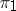andof, and every irreducible finite-dimensional representation ofis equivalent to a representation of the form. A description of the finite-dimensional representations of a simply-connected connected complex semi-simple Lie group can also be given in terms of the exponentials of finite-dimensional representations of its Lie algebra, and also by using the Gauss decomposition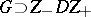of: Letbe a continuous function onsuch that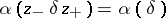for all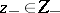,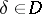,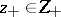, and suppose that the linear hull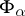of the functions,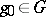, is finite-dimensional; then the formula,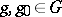,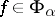, defines an irreducible finite-dimensional representation of, and all irreducible finite-dimensional representations ofcan be obtained in this way. Ifis a real semi-simple Lie group having complex form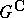, then every irreducible finite-dimensional representation ofis the restriction toof some unique irreducible finite-dimensional representation ofwhose matrix entries are analytic on(so that the theory of finite-dimensional representations of semi-simple connected Lie groups reduces essentially to that of compact Lie groups). A finite-dimensional representation of an arbitrary Lie group is real analytic; ifis a simply-connected Lie group, then there is a one-to-one correspondence between the finite-dimensional representations ofand of its Lie algebras (associating to a representation of the Lie group its differential; the inverse mapping associates to a representation of the Lie algebra its exponential). However, the classification of finite-dimensional representations of arbitrary Lie groups is far from being completely solved (1988), even for the special cases of,, and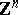,(see ). On the other hand, the irreducible finite-dimensional representationsof a connected Lie groupare known : They have the form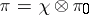, whereis a one-dimensional representation of(that is, essentially of its commutative quotient group by the commutator subgroup), and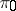is a finite-dimensional representation of the semi-simple quotient group ofby the maximal connected solvable normal subgroup of(see Levi–Mal'tsev decomposition).